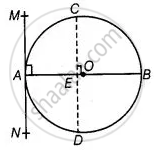# Prove that a diameter AB of a circle bisects all those chords which are parallel to the tangent at the point A. - Mathematics

Diagram
Sum

Prove that a diameter AB of a circle bisects all those chords which are parallel to the tangent at the point A.

#### Solution

Given, AB is a diameter of the circle.

A tangent is drawn from point A.

Draw a chord CD parallel to the tangent MAN.So, CD is a chord of the circle and OA is a radius of the circle

∠MAO = 90°   .....[Tangent at any point of a circle is perpendicular to the radius through the point of contact]

∠CEO = ∠MAO  .....[Corresponding angles]

∴ ∠CEO = 90°

Thus, OE bisects CD,  ......[Perpendicular from centre of circle to chord bisects the chord]

Similarly, the diameter AB bisects all.

Chords which are parallel to the tangent at the point A.

Concept: Concept of Circle - Centre, Radius, Diameter, Arc, Sector, Chord, Segment, Semicircle, Circumference, Interior and Exterior, Concentric Circles
Is there an error in this question or solution?

#### APPEARS IN

NCERT Mathematics Exemplar Class 10
Chapter 9 Circles
Exercise 9.3 | Q 10 | Page 108
Share